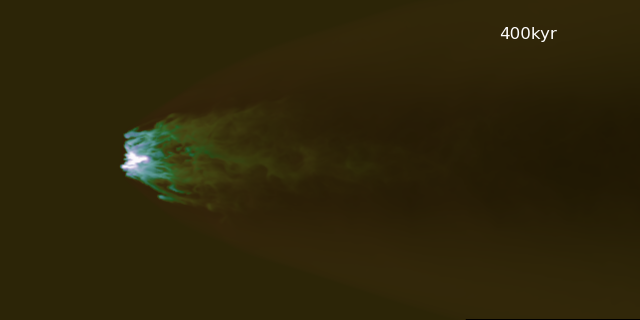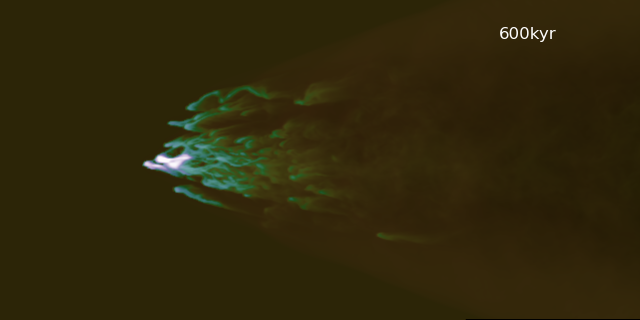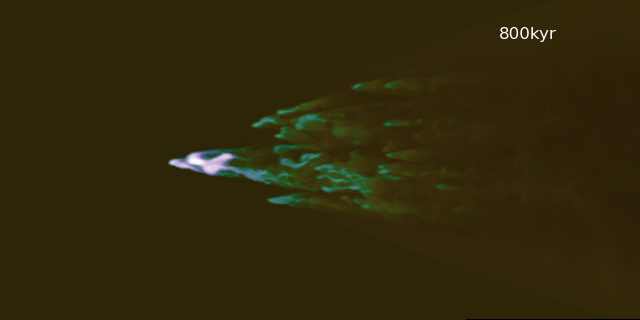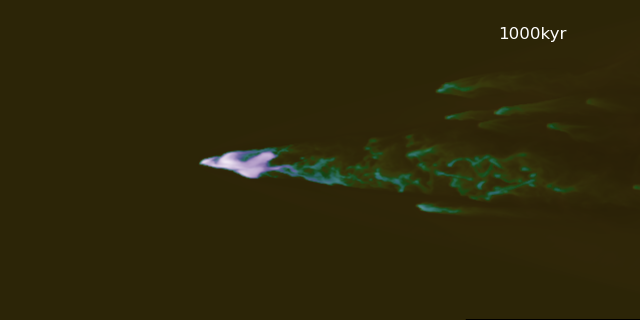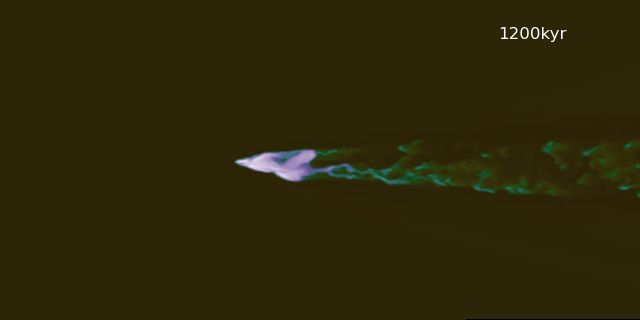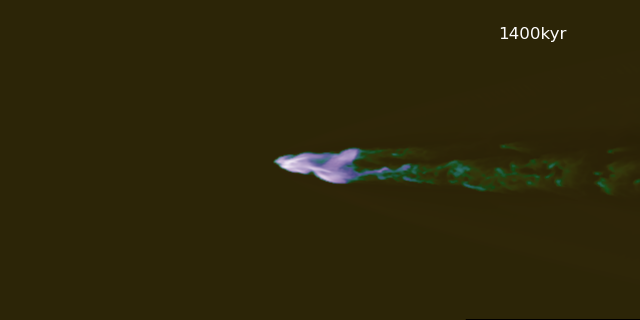Sphere Wind Simulation

January 25, 2016

Using the hot wind model discussed in the previous post, the following simulations show the evolution of a spherical cloud of cool, constant density gas. The sphere has a radius $R_{\mathrm{cloud}} = 5\,\mathrm{pc}$, and a density $n_h = 1.0 \,\mathrm{cm}^{-3}$. It is initially in pressure equilibrium with the hot wind, with wind parameters chosen at a distance of $R_{*} = 1\,\mathrm{kpc}$: $n_{wind} = 7.683798 \times 10^{-4}\,\mathrm{cm}^{-3}$, $v_{wind} = 1.229560\,\mathrm{km}\,\mathrm{s}^{-1}$, and $T_{wind} = 3.991611 \times 10^{6}\,\mathrm{K}$. The Mach number of the wind at this point is $M \approx 5.25$.

Below are snapshots from two simulations - one showing an adiabatic cloud, and one showing a cloud that is allowed to radiatively cool (the cooling sim also includes background heating from photoionization). Snapshots are shown every 200 kyr. The box has dimensions $50 \times 50 \times 100$ pc, and the cloud has an initial resolution of 32 cells / $R_{\mathrm{cloud}}$.

The following images show the evolution of an adiabatic simulation: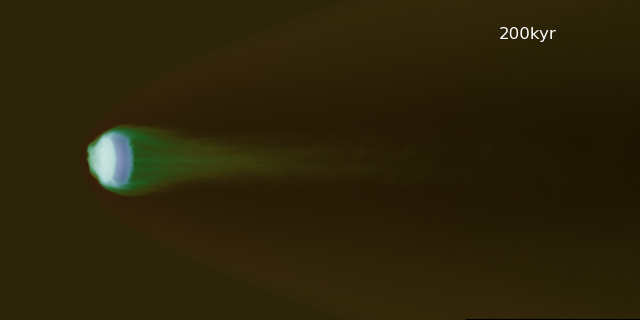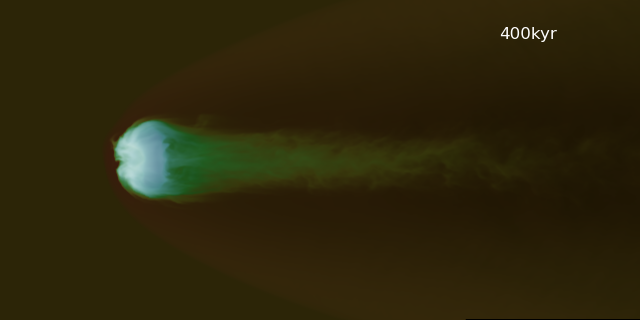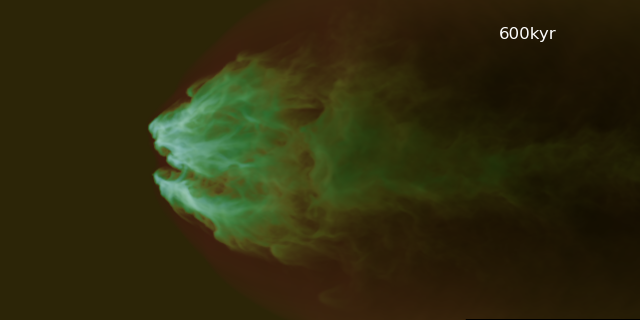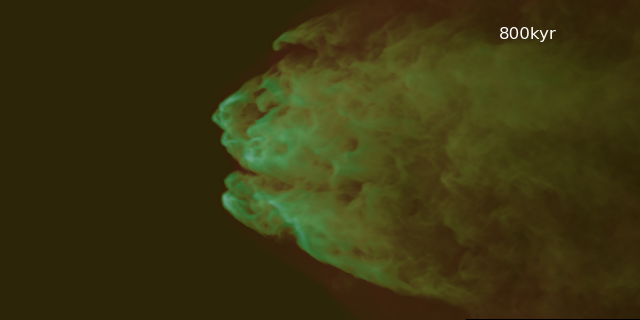This evolution can be contrasted with the radiatively cooling case: